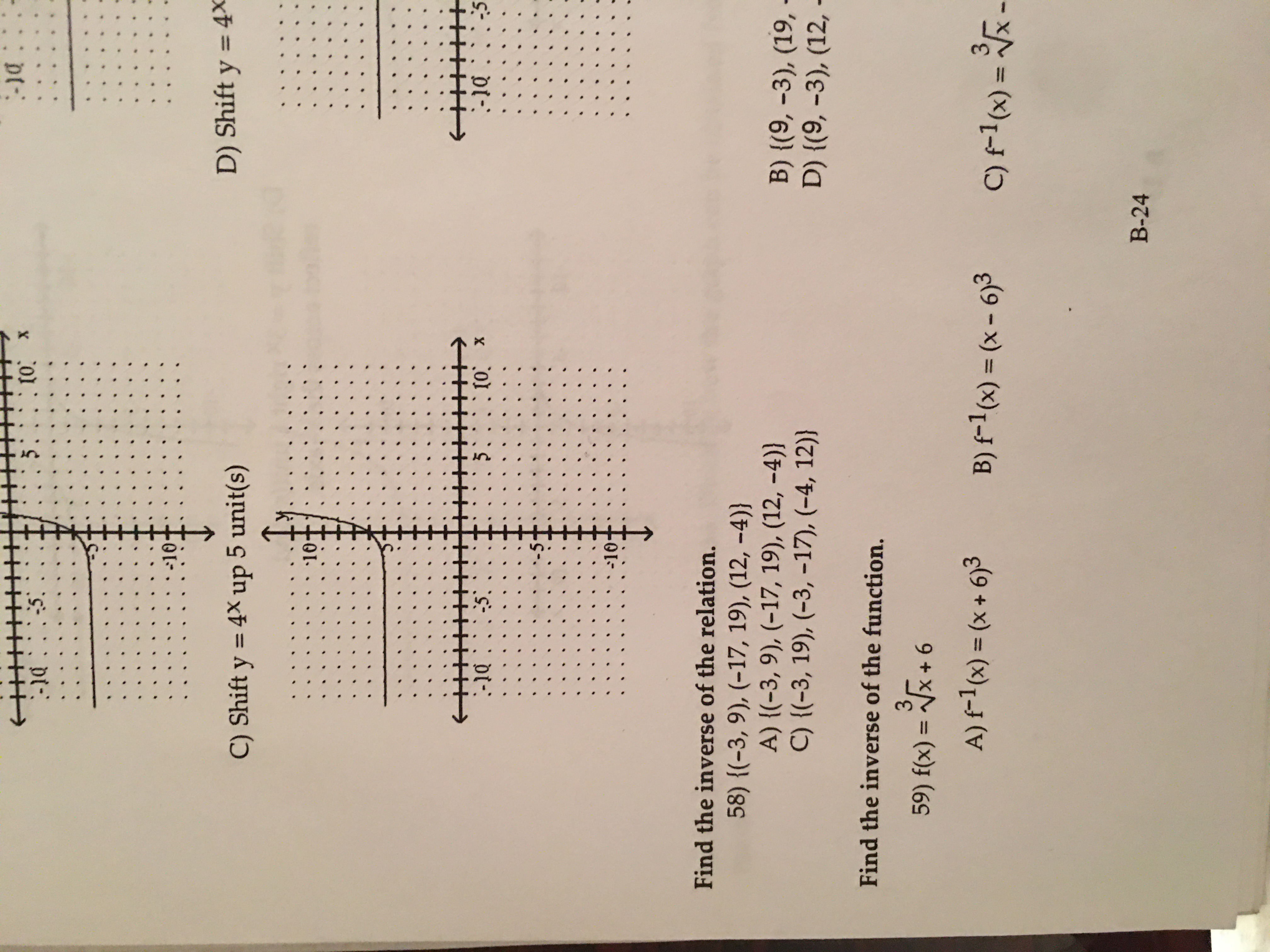# -1d: !5 t0 x-::-10D) Shift y = 4XC) Shift y 4X up 5 unit(s)105.:. (0 X-5-1:-10Find the inverse of the relation.58) {(-3, 9), (-17, 19), (12, -4))A) {(-3, 9), (-17, 19), (12, -4))C) {(-3, 19), (-3, -17), (-4, 12))B) {(9, -3), (19,D) {(9, -3), (12,Find the inverse of the function.359) f(x) = x +6A) f1(x) = (x+ 6)3B) f-1(x) = (x- 6)3C)1 (x)11В-24

Questionhelp_outlineImage Transcriptionclose-1d: ! 5 t0 x -:: -10 D) Shift y = 4X C) Shift y 4X up 5 unit(s) 10 5.:. (0 X -5 -1: -10 Find the inverse of the relation. 58) {(-3, 9), (-17, 19), (12, -4)) A) {(-3, 9), (-17, 19), (12, -4)) C) {(-3, 19), (-3, -17), (-4, 12)) B) {(9, -3), (19, D) {(9, -3), (12, Find the inverse of the function. 3 59) f(x) = x +6 A) f1(x) = (x+ 6)3 B) f-1(x) = (x- 6)3 C)1 (x) 11 В-24 fullscreen
check_circleExpert Solution
Step 1

The given function is

Step 2

Let

Step 3

Interchange the variables x and y and obtain the va...

### Want to see the full answer?

See Solution

#### Want to see this answer and more?

Solutions are written by subject experts who are available 24/7. Questions are typically answered within 1 hour*

See Solution
*Response times may vary by subject and question
Tagged in

### Functions